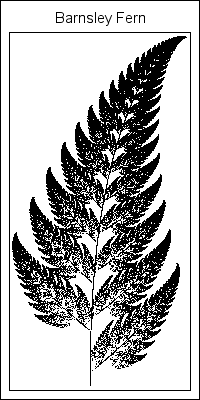Fractals. If you grew up in the 1980s or '90s and were interested in math and computers, chances are you played with computer generation of fractals. Who knows how many hours of computer time was spent computing Mandelbrot sets and Julia sets to ever-increasing resolutions?

When I was a kid, I realized that there were two kinds of fractals: the deterministic ones, such as the Mandelbrot set, and the probabilistic ones, such as the Barnsley fern. I spent most of my time generating the deterministic fractals, because they were simpler to understand for someone who knew little about probability or linear transformations.

Yet, I was always fascinated by how to create objects by using Michael Barnsley's iterated function systems (IFS). You can construct an IFS by defining some number of affine planar transformations, selecting one at random with some probability, and applying it to an initial point. By iteratively selecting and applying these affine transformations, the trajectory of the point traces out some fractal shape, such as the fern shown at the left. Now that I am older and know a little bit about probability and linear transformations, let's investigate how to construct Barnsley's fern in the SAS/IML matrix language. I invite DATA step programmers to create a similar program by using the SAS DATA step.

### Construct Barnsley's Fern in SAS

Before I began programming the IFS, I reminded myself how to construct an iterated function system. Take a few minutes to read about the underlying mathematics. You are then ready to construct the IFS for Barnsley's fern:

1. Create four affine transformations in the plane. Each affine transformation is of the form T(x) = A*x + b, where A is a 2x2 matrix, b is a 2x1 vector, and x is a 2x1 vector that represents a point in the plane.
2. Specify the probability of selecting each of the four transformations.
3. Iterate the discrete stochastic map by selecting an initial point and repeatedly doing the following a large number of times:
• Choose one of the transformations at random, using the given (unequal) probabilities.
• Apply the chosen transformation to the current point to obtain a new (image) point.
4. Plot the trajectory (or orbit) of the initial point.
Under certain conditions on the transformations (they need to be contracting), the orbit of the initial point is attracted to a fractal set.

The following SAS/IML program implements an iterated function system for creating Barnsley's fern. The SGPLOT procedure is used to plot the trajectory. The main programming details are that the RANDGEN subroutine is called to generate random numbers from the "Table" distribution, and the SHAPE function is used to convert a row of coefficients into a 2x2 matrix. The SAS/IML matrix-vector operations are used to apply each affine transformation.

```/* http://en.wikipedia.org/wiki/Barnsley_fern The Barnsley fern is created with four affine transformations: T1(x,y) = [ 0 0 ] * [x] with prob=0.01 [ 0 0.16] [y]   T2(x,y) = [ 0.85 0.04] * [x] + [0 ] with prob=0.85 [-0.04 0.85] [y] [1.6]   T3(x,y) = [ 0.2 -0.26] * [x] + [0 ] with prob=0.07 [ 0.23 0.22] [y] [1.6]   T4(x,y) = [-0.15 0.28] * [x] + [0 ] with prob=0.07 [ 0.26 0.24] [y] [0.44] */ proc iml; /* 1. Each row is a 2x2 linear transformation */ L = {0 0 0 0.16, /* L1 */ 0.85 0.04 -0.04 0.85, /* L2 */ 0.2 -0.26 0.23 0.22, /* L3 */ -0.15 0.28 0.26 0.24}; /* L4 */   /* ... and each row is a translation vector */ B = {0 0, /* B1 */ 0 1.6, /* B2 */ 0 1.6, /* B3 */ 0 0.44}; /* B4 */ /* For convenience, transpose the L and B matrices */ L = L`; B = B`;   /* 2. The transformations are of the form T(x) = A*x + b where A and b are chosen randomly from the columns of L and B. Specify probability of each transformation. */ prob = {0.01 0.85 0.07 0.07};   /* 3. iterate the discrete stochastic map */ N = 1e5; /* number of iterations */ x = j(2,N); x[,1] = {0, 2}; /* initial point */   do i = 2 to N; call randgen(k, "Table", prob); /* k is a number 1,2,3 or 4 */ x[,i] = shape(L[,k], 2)*x[,i-1] + B[,k]; /* apply transformation to x[i-1] */ end;   /* 4. plot the iteration history */ y = x`; create IFS from y[c={"x" "y"}]; append from y; close IFS; quit;   ods graphics / width=200px height=400px; proc sgplot data=IFS; title "Barnsley Fern"; scatter x=x y=y / markerattrs=(size=1); yaxis display=none; xaxis display=none; run;```

The algorithm creates the image that appears at the beginning of this article.

### The holiday challenge: Construct an IFS for a Christmas tree

The cool thing about iterated function systems is that the affine transformations and the probabilities are actually parameters that you can vary. If you choose a different set of affine transformations and/or a different set of probabilities, you get a different fractal attractor. You can find Web sites that show other ferns, leaves, and branches that result from using different parameter values.

Last year during the holiday season I published some attempts to construct a Christmas tree by using the SAS DATA step. It was fun to see contributions from many SAS programmers, including a menorah! This year I issue the following challenge: can you find parameter values for an IFS that create a Christmas tree image?

I will publish my program that creates a fractal Christmas tree in a few days, so start working on your ideas! You can submit your program as a comment to my solution. Not into Christmas trees? How about a Koch snowflake instead?

Share